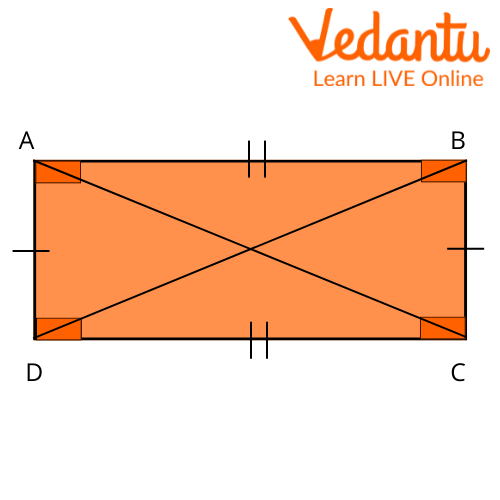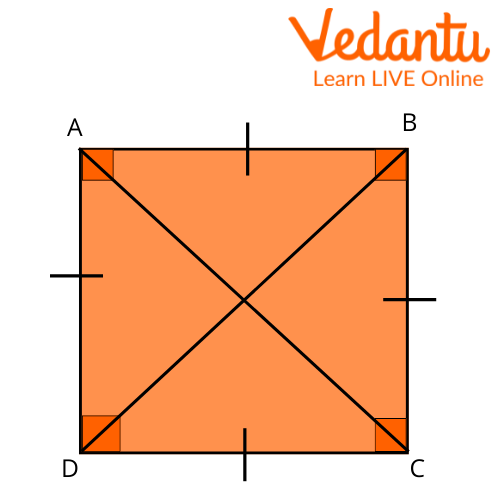Courses
Courses for Kids
Free study material
Free LIVE classes
More

# How to Construct a Parallelogram?LIVE
Join Vedantu’s FREE Mastercalss

## What is a Parallelogram?

If you're struggling with geometry, don't worry. With this step-by-step guide, you'll be able to know how to make a parallelogram or construct it in no time! Just remember the basic definition of a parallelogram - It is a special kind of quadrilateral that is formed by parallel lines. The angle between the adjacent sides of a parallelogram may vary, but the opposite sides need to be parallel for it to be a parallelogram.

So, it can also be said that a parallelogram is a quadrilateral. A parallelogram has two pairs of parallel lines, called the bases, while the other pair is a set of diagonals that intersect at their midpoints. In this article, we will look at parallelograms and how to draw a parallelogram.Parallelogram

## Properties of a Parallelogram

Following are the Properties of a Parallelogram:

A parallelogram can be identified using certain simple properties. Examine the following parallelogram PQTR in relation to the properties listed below.Parallelogram PQTR

The following properties can help us identify and differentiate a parallelogram:

• The opposite sides of a parallelogram are parallel. Here, $PQ \| RT$ and $PR \| Q T$.

• The opposite sides of a parallelogram are equal. Here, $P Q=R T$ and $P R=QT$

• The opposite angles of a parallelogram are equal. Here, $\angle P=\angle T$ and $\angle Q=\angle R$

• The diagonals of a parallelogram bisect each other. Here, RE = EQ and $P E=E T$

• Same-side interior angles supplement each other. Here, $\angle P R T+$ $\angle R T Q=180^{\circ}, \angle R T Q+\angle T Q P=180^{\circ}, \angle T Q P+\angle Q P R=180^{\circ}, \angle Q P R+$ $\angle P R T=180^{\circ}$

• The diagonals divide the parallelogram into two congruent triangles. Here, $\triangle \mathrm{RPQ}$ is congruent to $\triangle \mathrm{RTQ}$, and $\triangle \mathrm{RPT}$ is congruent to $\triangle Q T P$

A quadrilateral with two opposite pairs of parallel sides is known as a parallelogram. A parallelogram's opposite sides are equal in length, and its opposite angles are equal in size. Furthermore, the interior angles on the same transversal side are additional. And that is why we call a parallelogram a quadrilateral.## Can a Square be a Parallelogram?

A parallelogram having four equal sides and four right angles is called a square.Square ABCD

Examine the square ABCD to see how it relates to the following qualities. A square contains:

• Four equal sides. Here, $A B=B C=C D=D A$

• Four right angles. Here, $\angle \mathrm{A}=\angle \mathrm{B}=\angle \mathrm{C}=\angle \mathrm{D}=90^{\circ}$

• Two pairs of parallel sides. Here, $A B \| D C$ and $A D|| B C$

• Two equal diagonals. Here, $A C=B D$

• Diagonals that are perpendicular to each other. Here, $A C \perp B D$

• Diagonals that bisect each other.

Therefore, from the above properties, it can be said that a square is a parallelogram.

## Construct a Parallelogram

Construct a Parallelogram When Two Consecutive Sides and the Included Angle are given:

• Step 1: Construct a line segment $A B=4 \mathrm{~cm}$.

• Step 2: Construct a 60-degree angle at point $A$.

• Step 3: Construct a line segment $A D=5 \mathrm{~cm}$ on the other arm of the angle.

• Step 4: Then, place the sharp point of $5 \mathrm{~cm}$ above $B$

• Step 5: Stretch your compasses to $4 \mathrm{~cm}$, place the sharp end at D and draw an arc to intersect the arc drawn in step 2.Draw a Parallelogram

• Step 6: Label the intersecting point $C$.

• Step 7: Join $C$ to $D$ and $B$ to $C$ to form the parallelogram $A B C D$.

## Practice Problems

Q 1. Construct a parallelogram whose diagonal is 5.4 cm and 6.2 and an angle between them is 70.

Q 2. Construct a parallelogram when one of its side is $4 \mathrm{~cm}$ and its two diagonal are $5.6 \mathrm{~cm}$ and $7 \mathrm{~cm}$. Measure the other side.

## Summary

A parallelogram is a four-sided figure with two pairs of parallel sides. Then we saw the properties of a parallelogram, and we came to know that a square is a parallelogram. To construct a parallelogram, you will need a scale and a compass.

First, use the compass to draw two arcs that intersect at two points. Then, use the scale to connect the points of intersection. Finally, use the compass to draw two more arcs that intersect at two additional points. Connect these points as well, and you will have created a parallelogram. We have now seen how to construct a parallelogram given certain conditions.

Last updated date: 20th Sep 2023
Total views: 114.3k
Views today: 2.14k

## FAQs on How to Construct a Parallelogram?

1. What are real-life examples of parallelograms?

Most desks and tables are rectangular or square in shape. A rectangular table has two sets of parallel, equal-length sides. As a result, a rectangular is a parallelogram as well. As a result, rectangular tables are a common example of parallelogram-shaped objects in daily life.

2. What is the difference between a parallelogram and a quadrilateral, and is a trapezoid a parallelogram?

All parallelograms are quadrilaterals, but all quadrilaterals aren't always parallelograms. For instance, a trapezoid is a quadrilateral; however not a parallelogram, considering that all opposite faces of the trapezoid aren't parallel to every other. A trapezoid has only one pair of opposite sides parallel to each other. Also, a trapezoid does not have contrary aspects identical to every other. Hence, it's far from a quadrilateral, but not a parallelogram.

3. What is the formula for the perimeter of a parallelogram?

The opposite sides of a parallelogram are parallel to each other, and the parallelogram perimeter is defined as two times the base and height.

Perimeter of a parallelogram, P = 2(a+b). Here, a and b are the base and the height.